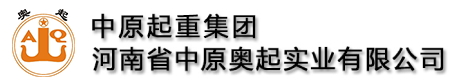全国统一服务热线:13782575673

# 花架式龙门吊大车主梁设计计算

### 来源：本站   发布时间：2021-03-16 03:06:49  点击量：31

①设计计算主梁梁高

运行冲击系数取φ4=1.24;起升冲击系数取φ2=1.22;

截面腹板厚度初选：δ=δ1+δ2=28mm;δ1=16mm;δ2=12mm;

采用Q235B材料，许用应力[σ]=1.40×105kN/m2;采用Q345B材料，许用应力[σ]=2.30×105kN/m2;弹性模量E=2.1×108kN/m2;许用刚度初选β=700;腹板加劲板重量比腹板重量α=1/3;

走台栏杆轨道机械电气设备重量与主梁重量之比λ=0.2;材料比重γ=78.5kN/m3;

C1将小车轮压转化为跨中集中力时计算弯矩的换算系数C1≈1;

C2将小车轮压转化为跨中载荷计算挠度的换算系数主梁采用Q235B材料，主梁梁高计算为：[Q]

根据强度控制梁高的公式计算梁高，先求出K1。圆整取hq=3300mm。

强度为控制条件，采用Q235材料，工作级别为A5;为了减轻主梁重量，节约材料，主梁的材料改为Q345，其梁高计算为刚度为控制条件。圆整取hg=2750。

(3300-2750)/3100=17.7%

由上计算可知，对A5以下级别的起重机，当强度为控制条件时，改用Q345材料可以节约钢材，减轻主梁的重量约5%～8%。

②初步确定主梁截面面积取经济梁高盖板与腹板截面积之比?，不设走台，把主梁兼做走台;上盖板δ3=24，下盖板δ4=20。

上盖板的截面积尺寸?

上盖板的宽度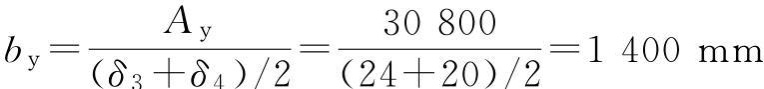by=1400mm作为主腹板和副腹板之间的净宽距离。

③确定大车主梁承压梁的梁高

将主梁跨端的承压梁上下盖板、主副腹板加强，厚度全部等于24mm，采用Q235-B材料，根据主梁设计计算和用户给定参数得以下数据。

φ2=1.22;φ4=1.24;GXC=580000N;Gq=1380000N;Q=3000000N;B1=2018mm;B2=1578mm;δ1=δ2=δ3=δ4=24mm;b0=1430mm;[τ]=80MPa;Ix=14.56×109mm4。

参考同类欧式大车主梁的承压梁高，取h1=800mm，则Sx=19987614mm3(图4-53)。④主梁的筋板布置

主梁采取全偏轨梁，对腹板，当160<2750/16=172时，按高腹板局部稳定性原则，多设置几道纵向加劲杆，第一道高度宜不大于500mm，其他道高度宜不大于800mm。第一道设在距上盖板500mm处，第二道设在距上盖板1300mm处，第三道设在距上盖板2100mm处(图4-53)。

当上下盖板较宽时，宜设置一道或多道纵向加劲杆。所划分出来的区格宽度c不大于50δ。

b0=1400mm;δ3=24，δ4=20;b0/δ3=58>50，b0/δ4=70>50

在上下盖板分别设置一道纵向加劲杆(图4-53)。

⑤对主梁进行Ⅲ类载荷组合验算两腹板中心线间距b1=1400mm，主梁高度h0=2750mm;运行冲击系数取φ4=1.24;起升冲击系数取φ2=1.22;大车加减速a=0.1m/s2;额定起升量Q=3000000N;小车重量GXC=580000N;小车基距b=4400mm;小车跨距S=33500mm。大车半个桥架的重量Gq=565000N(主梁+走台);

主梁走台自重均布载荷：均布载荷在跨中引起的最大弯矩：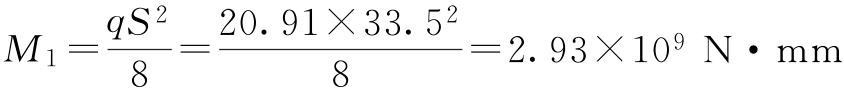作用于一根主梁上的载荷：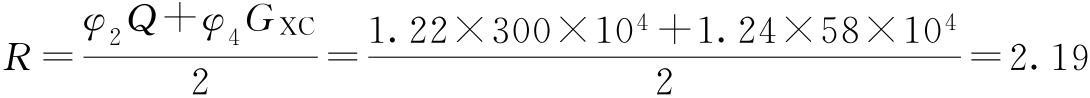在设计小车时，得：a1=2300mm，a2=2100mm(图4-54)。

主梁惯性矩：Ix=2.081×1011mm4;y1=1521mm;y2=1273mm;Iy=6.05×1010mm4;x1=820mm;x2=708mm;弹性模量E=2.1×108MPa。

小车轮压：a.主梁垂直静挠度垂直刚度满足设计要求。

b.垂直平面内的应力计算

集中力引起的跨中最大弯矩：垂直方向主梁跨中截面正应力：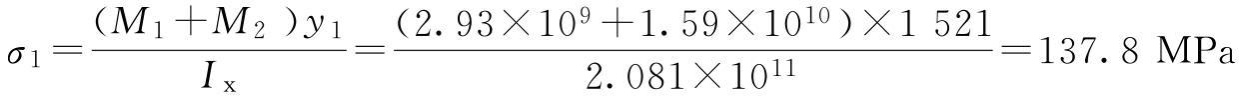c、水平平面内的应力计算

均布惯性载荷：均布惯性载荷跨中产生的最大弯矩：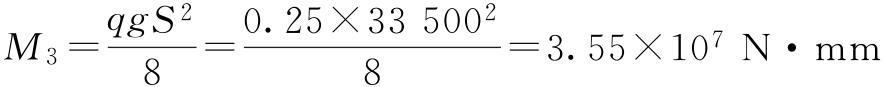小车对主梁的集中惯性载荷：

Pg=1.5(GXC+Q)×0.1×0.5×a=1.5×(58+300)×104×0.1×0.5×0.1=2.69×104N

集中惯性载荷引起的跨中最大弯矩：水平方向主梁跨中截面正应力：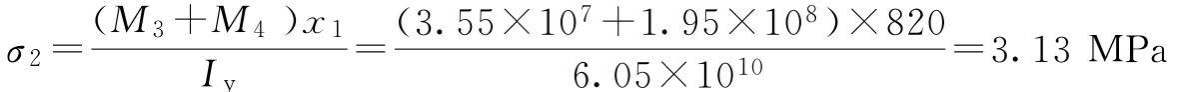综合应力：σ=1.15(σ1+σ2)=1.15(137.8+3.13)=162MPa≤230MPa

强度满足设计要求，1.15是考虑主梁跨中的约束扭转和约束弯曲的影响。

水平挠度：水平刚度满足设计要求。